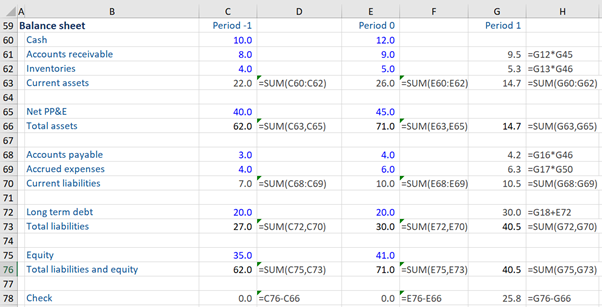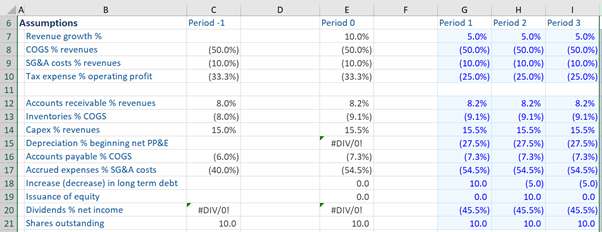## What is “Forecasting Balance Sheet Line Items”?

As part of the financial modeling process, analysts will need to forecast the company’s balance sheet. This will help show how the assets and liabilities are ‘moving’ through the forecasted period. Setting up a model to do this is typically done line-by-line once assumptions have been made for future growth.

Here is a reminder of the four steps to build a financial model:

1.      Input publicly available (or private) historical data – the financial model’s projections are typically based on the historical financial performance of the company

2.      Construct ratios and statistics predicated on the historical data

3.      Make assumptions based on the above two steps

4.      Forecast balance sheet line items using these assumptions

## Key Learning Points

• Forecasting balance sheet line items will be based on the set of assumptions that an analyst makes about the company’s anticipated performance in future business conditions
• A good modeler, when attempting to forecast the line items of a balance sheet statement, should initially build the first forecast period only (i.e. period 1) and check it thoroughly before copying it across to the later years (i.e. period 2 and beyond)

Often it is helpful to build the income statement forecasts first and then complete the balance sheet forecasts. The balance sheet forecasts will then be linked to the cash flow (along with the income statement) to give a full view across all three financial statements. Part of the balance sheet forecasting will usually be completed using BASE analysis.

## Assumptions, Subtotals, and Checking

The process of forecasting the balance sheet line items is very similar to that of forecasting the income statement line items.

The first step involves calculating subtotals (of historical periods) wherever applicable in the balance sheet so that the forecasts can be compared to the historical performance. This is usually to sum up: current assets, total assets, current liabilities, total liabilities, and total liabilities and equity. This is done for the historical period and then can be copied across to forecast periods. It is important to not rebuild subtotals through the model as it can allow man-made errors and potential typing errors to alter the forecasts.

The example below will go through the line-by-line steps. The important first step is to build the first forecast year before copying it across to later years. It might seem easier to create a line and then drag it over for future years, but this may mean that any corrections made are not uniformly applied to all the years. Therefore it is sensible to build one full year of forecasts, check for accuracy (and that the balance sheet ‘balances’), and then apply them to subsequent years. Occasionally, each line must be copied across individually, but copytable formulas are much more commonly used.

## Example – Forecasting Balance Sheet Line Items

Given below is an example of how to build a balance sheet. Here, we are forecasting the line items of period 1 (i.e. the first forecast period) only.

To start, we are given the balance sheet – historical period (period -1 and period 0). We first need to fill in the subtotals for the historical periods i.e. current assets, total assets, current liabilities, total liabilities, and total liabilities and equity. Thereafter, we need to use the assumptions to help produce our forecast figures.

Two items that we will not look at here are the forecast period’s Net PP&E and Equity. At this stage, it’s sufficient to know that later on, we need to do calculations for them. These are usually forecast using BASE analysis and then linked back into the models.

Once we have completed the subtotals and checked them,  we can copy them to the next historical period.It is important to check that the balance sheet ‘balances’. The modeler can add a ‘Check’ line which simply takes the sum of total liabilities and equity and minus the total assets. If the balance sheet balances, this should come to zero, as it does here in our example. Again once working, this can be copied across to subsequent years.

Now we go to the next step – that is looking at the forecast periods.The table above shows all the assumptions for the upcoming three years. These can be set up in various ways and aren’t always uniform as companies may, for example, provide more detail in a certain area which can be factored in. Also, there may be variations in forecasting techniques. For example, capex may be forecast as a % of sales or it could be done as a % of assets, depending on how the model is put together. It is important to glean all the available information from the company and use it in the assumptions. Often if a data point is provided by a company (e.g. a sales breakdown by region) then investors will want to know this has been considered and factored into a model.

Looking back to the balance sheet, let’s consider it line by line. Firstly, we leave the cash for the period 1 forecast period blank, because we will need the cash flow statement to fill it. Then we move on to accounts receivable. To forecast this, we go to the assumptions and find the accounts receivable % revenues assumption – which is 8.2% for period 1. So we can multiply this assumption by revenues forecast for period 1 and can see from this equals 9.5.We do the same thing for inventories, go to the assumptions and find the inventories % COGS for period 1 (9.1%). We multiply this assumption by the COGS forecast for period 1 to get the inventories forecast for period 1. It is important when building the model to make sure the formula is linking to the correct year or time period. Otherwise, a model can look to be fully functioning but the balance sheet is unlikely to balance and this can hold up the process.

Next, we go to Net PP&E. We will leave this for now as it usually forms part of the BASE analysis.

To compute the account payables, we go to assumptions and look at the accounts payable % COGS assumption for period 1 (7.3%). This we will multiply by COGS forecast for period 1 to obtain the accounts payable for period 1. This same approach is used for accrued expenses.

To obtain the long-term debt forecast for period 1, we go up to our assumptions again and find that for period 1 the assumption is an increase or decrease in long-term debt of 10% for period 1. Now we need to add this to the last historical period’s long-term debt of 20. In this example, it then gives a long-term debt of 30.

We will leave calculating the equity for period 1 out for the moment (as already stated above). So our balance sheet for period 1 has been done or built apart from cash, net equity, and Net PP&E. We can quickly check if our balance sheet balances. Unfortunately, it is unbalanced by 25.8 in period 1. This is because we have missed these three items in the forecasts.

To conclude, when the calculations for these three items are done, we will put these in our balance sheet and then the balance sheet balances not only in the historical period but also in period 1(i.e. in the first forecast period).

### Conclusion

Forecasting the balance sheet is a straightforward job if done carefully and using the steps to minimize the chance of accidental errors. The balance sheet forecasts can be completed once the cash flow and BASE analysis are done. Then they are linked together – this can risk causing circular references if not done carefully so checks must be made afterward that all the assumptions and forecasts are linked up appropriately.

Quite often investors will want to know how much cash or debt is forecast to build up over the forecasted period so a forecasted balance sheet is a very useful tool to have. The more detail and effort that is put into the building of the forecasts then the more valuable the model tends to be!